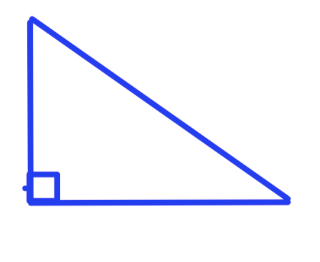### Pythagaros

Thursday, February 26, 2009The first artifact is a a right angle triangle, also known as 'R.A.T'. The square in the corner is the right angle, and it's not called a right triangle because it's on the right side (in this case its not on the right!) The right angle is an angle of 90 degrees. The triangle is made up of legs: A and B, and the longest side of the triangle or the part of the triangle that is diagonal from the right angle is called the hypotenuse. The acute angles are going to be Theta and Beta. Theta and Beta are called complimentary angles because together they form a 90 degree angle.This second artifact is a square, this is a special type of rectangle. A square has 4 equal sides. Those 4 equal sides are called lines of symmetry. A square is made up of 90° angles, one at each corner. So the square makes 360°because 4 x 90° = 360°. In this picture, there are 2 right angle triangles.

This third artifact is the Phythagorean Theoem. This means that, if you know side "a" and side "b", then all you do is square them. So, you have to add them together, which leaves you the c" squared. Then find the squar root of the answer from your calculator, that will give you the length of side "c".This is a statue of Pythagoras. He is the great greek man that made the Pythgorean Theorem and many more. He was the first known westerner to say that the Earth revolves around the sun. He was the only westerner to be allowed in pyramids, only one to speak fluent Egyptian language.

Pythagoras: The greatest genius ever lived. He is a "uber" smart mathematician, he was a Greek geek. It wasn't for sure that he was really alive or even existed.

Legs: These are connected to create the right angle of the right angle triangle a.k.a sides "A" and "B". They are also smaller than the hypotenuse.
Hypotenuse: The hypotenuse is the longest side of the right angle triangle, its the opposite side of the right angle also.
R.A.T: stands for Right Angle Triangle.
Greek : A person that from ancient modern Greece, also means that you lives or was born in Greece.
Theorem: A theoretical proposition, statement, or formula, something to be proved from other formulas.

In this problem that Mr.Harbeck gave us, we had to find the base of the triangle. This picture explains how I solved 'c'.

Problem#2:First, you need to find out how long each side of the square is. Then that will give you side "A" and "B" for the triangles since they're both the same length. Okay so, like a normal question find the length of the hypotenuse, which is 'c'. Once you have gott that you can now label the triangle and add up all the sides to find the perimeter.